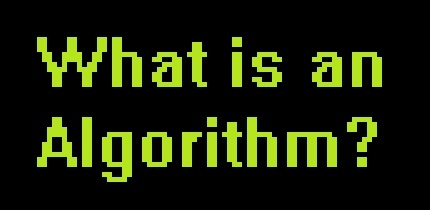Algorithms Quiz
3 years ago
fitzmrjohnson
Save
Edit
Host a game
Live GameLive
Homework
Solo Practice
Practice20 QuestionsShow answers
• Question 1
30 seconds
Q. What is an Algorithm?
A chart showing the flow of a series of events
Step-by-step instructions used to solve a problem
A decision arrived at by following instructions
A computer program that follows a chart
• Question 2
30 seconds
Q. What is a flowchart?
A text-based way of designing an algorithm
A specific programming language
A diagram that represents a set of instructions
A bullet point list of instructions
• Question 3
30 seconds
Q. What algorithm do we use when we're looking for the Post Office?
A set of directions
A recipe
A list of instructions
• Question 4
30 seconds
Q. Programming languages give computers instructions
True
False
• Question 5
30 seconds
Q. What algorithm do we use when we're building a wardrobe?
A set of directions
A recipe
A list of instructions
• Question 6
30 seconds
Q. We can show the sequence of steps in an algorithm in a structural diagram called a flow chart.
True
False
• Question 7
30 seconds
Q. Only top software programmers can write an algorithm.
True
False
• Question 8
30 seconds
Q. What algorithm do we use when we're making a cake?
A set of directions
A recipe
A list of instructions
• Question 9
30 seconds
Q. When you write an algorithm the order of the instructions is very important.
True
False
• Question 10
30 seconds
Q. What should be considered when designing an algorithm?
If the correct hardware is being used
If the correct software is being used
If there is more than one way of solving the problem
• Question 11
30 seconds
Q. In a flowchart how are symbols connected?
Symbols do not get connected together in a flowchart
With lines and an arrow to show the direction of flow
With dashed lines and numbers
With solid lines to link events
• Question 12
30 seconds
Q. When can algorithms be used?
Only with computers
Only when programming
Only with flowcharts
Any time to design solutions to problems
• Question 13
30 secondsQ.
This is a flowchart
This is pseudocode
This is codechart
This is decision chart
• Question 14
30 seconds
Q. An algorithm ...
... illustrates some steps, in no particular order, and following no logical reasoning
... illustrates a series steps in a particular order, following logical reasoning
... illustrates some random shapes in a flowchart but in a set order
... illustrates series of decisions needed to bake a cake
• Question 15
30 secondsQ. What is this symbol used for in a flowchart?
A decision/question
A task to carry out (process)
Input or Output data
Start / Stop
• Question 16
30 secondsQ. What is this symbol used for in a flowchart?
A decision/question
Start / Stop
Input or Output data
• Question 17
30 secondsQ. What step is missing?

Divide the number by 2
Multiply the number by 2
Multiple the number by 3
Divide the number by 3
• Question 18
30 seconds
Q. What does an arrow represent in a flow chart
Decision making
Data Flow
Start
Stop
• Question 19
30 seconds
Q. What shape in the flow chart represents a decision
Square
Diamond
Rectangle
Oval
• Question 20
30 seconds
Q. If something needs to be done (processing) what flowchart symbol do we use?